# US8004320B2 - Frequency synthesizer, frequency prescaler thereof, and frequency synthesizing method thereof - Google Patents

Frequency synthesizer, frequency prescaler thereof, and frequency synthesizing method thereof Download PDF

## Info

Publication number
US8004320B2
US8004320B2 US12/270,860 US27086008A US8004320B2 US 8004320 B2 US8004320 B2 US 8004320B2 US 27086008 A US27086008 A US 27086008A US 8004320 B2 US8004320 B2 US 8004320B2
Authority
US
United States
Prior art keywords
signal
frequency
positive
negative
output terminal
Prior art date
Legal status (The legal status is an assumption and is not a legal conclusion. Google has not performed a legal analysis and makes no representation as to the accuracy of the status listed.)
Active, expires
Application number
US12/270,860
Other versions
US20100085085A1 (en
Inventor
Tzu-Cheng Yang
Current Assignee (The listed assignees may be inaccurate. Google has not performed a legal analysis and makes no representation or warranty as to the accuracy of the list.)
Novatek Microelectronics Corp
Original Assignee
Novatek Microelectronics Corp
Priority date (The priority date is an assumption and is not a legal conclusion. Google has not performed a legal analysis and makes no representation as to the accuracy of the date listed.)
Filing date
Publication date
Priority claimed from TW97138272 external-priority
Application filed by Novatek Microelectronics Corp filed Critical Novatek Microelectronics Corp
Assigned to NOVATEK MICROELECTRONICS CORP. reassignment NOVATEK MICROELECTRONICS CORP. ASSIGNMENT OF ASSIGNORS INTEREST (SEE DOCUMENT FOR DETAILS). Assignors: YANG, TZU-CHENG
Publication of US20100085085A1 publication Critical patent/US20100085085A1/en
Application granted granted Critical
Publication of US8004320B2 publication Critical patent/US8004320B2/en
Active legal-status Critical Current

• 230000002194 synthesizing Effects 0.000 title description 26
• 238000010586 diagram Methods 0.000 description 20
• 230000001960 triggered Effects 0.000 description 10
• 101710004041 PCMP-A2 Proteins 0.000 description 6
• 230000000051 modifying Effects 0.000 description 6
• 101700001709 ubx2 Proteins 0.000 description 6
• 101700052479 SUL2 Proteins 0.000 description 4
• 230000000875 corresponding Effects 0.000 description 4
• 230000004048 modification Effects 0.000 description 4
• 238000006011 modification reaction Methods 0.000 description 4

## Images

•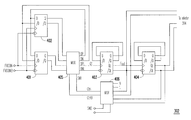•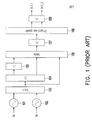•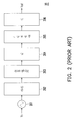•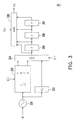•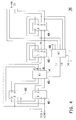•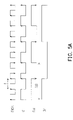•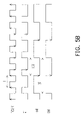•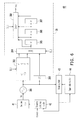•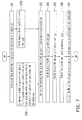## Classifications

• HELECTRICITY
• H03BASIC ELECTRONIC CIRCUITRY
• H03LAUTOMATIC CONTROL, STARTING, SYNCHRONISATION, OR STABILISATION OF GENERATORS OF ELECTRONIC OSCILLATIONS OR PULSES
• H03L7/00Automatic control of frequency or phase; Synchronisation
• H03L7/06Automatic control of frequency or phase; Synchronisation using a reference signal applied to a frequency- or phase-locked loop
• H03L7/16Indirect frequency synthesis, i.e. generating a desired one of a number of predetermined frequencies using a frequency- or phase-locked loop
• H03L7/18Indirect frequency synthesis, i.e. generating a desired one of a number of predetermined frequencies using a frequency- or phase-locked loop using a frequency divider or counter in the loop
• H03L7/197Indirect frequency synthesis, i.e. generating a desired one of a number of predetermined frequencies using a frequency- or phase-locked loop using a frequency divider or counter in the loop a time difference being used for locking the loop, the counter counting between numbers which are variable in time or the frequency divider dividing by a factor variable in time, e.g. for obtaining fractional frequency division
• H03L7/1974Indirect frequency synthesis, i.e. generating a desired one of a number of predetermined frequencies using a frequency- or phase-locked loop using a frequency divider or counter in the loop a time difference being used for locking the loop, the counter counting between numbers which are variable in time or the frequency divider dividing by a factor variable in time, e.g. for obtaining fractional frequency division for fractional frequency division
• H03L7/1976Indirect frequency synthesis, i.e. generating a desired one of a number of predetermined frequencies using a frequency- or phase-locked loop using a frequency divider or counter in the loop a time difference being used for locking the loop, the counter counting between numbers which are variable in time or the frequency divider dividing by a factor variable in time, e.g. for obtaining fractional frequency division for fractional frequency division using a phase accumulator for controlling the counter or frequency divider

## Abstract

A frequency synthesizer is provided, including a voltage-controlled oscillator (VCO), a frequency prescaler, a divide-by-2.5 circuit, and a selector. The VCO determine the frequency of a first signal according to an input voltage. The frequency prescaler determines the frequency of a second signal to be the frequency of the first signal divided by 3, 3.5, or 4 according to a first selection signal, and the frequency prescaler also determines the frequency of a third signal to be the frequency of the first signal divided by 6, 7, or 8 according to the first selection signal. The divide-by-2.5 circuit generates a fourth signal, wherein the frequency of the fourth signal is the frequency of the first signal divided by 2.5. The selector selects one of the second signal, the third signal, and the fourth signal as a fifth signal according to a second selection signal.

## Description

CROSS-REFERENCE TO RELATED APPLICATION
This application claims the priority benefit of Taiwan application serial no. 97138272, filed on Oct. 3, 2008. The entirety of the above-mentioned patent application is hereby incorporated by reference herein and made a part of this specification.
BACKGROUND OF THE INVENTION
1. Field of the Invention
The present invention generally relates to a frequency synthesizer, a frequency prescaler thereof, and a frequency synthesizing method thereof, and more particularly, to a frequency synthesizer with low circuit complexity, low power consumption, and broad bandwidth, a frequency prescaler thereof, and a frequency synthesizing method thereof.
2. Description of Related Art
A frequency synthesizer is a device for outputting signals of different frequencies, and is usually applied in the tuner of a broadband communication receiver. When the communication receiver needs to generate signals of different frequencies, the frequency synthesizer provides a high-frequency signal S1 and several signals S2˜SK of lower frequencies, wherein the frequency of the signal S1 is multiples of the frequencies of the signals S2˜SK.
FIG. 1 is a system block diagram of a conventional frequency synthesizer 10. Referring to FIG. 1, the conventional frequency synthesizer 10 includes at least two voltage-controlled oscillators (VCOs) 101 and 102, selectors 103 and 106, a divide-by-3.5 circuit 104, divide-by-2 circuits 105, 107, and 109, and an orthogonal signal separator 108.
The VCOs 101 and 102 are two different VCOs. The VCOs 101 and 102 receive an input voltage Vin and output signals of different frequencies according to the input voltage Vin. The selector 103 is coupled to the VCOs 101 and 102, and selects an output signal of the VCOs 101 and 102 as its output signal. Through the operations of the VCOs 101 and 102 and the selector 103, the selector 103 can output a signal having frequency between 1.8 GHz and 3.3 GHz.
The divide-by-3.5 circuit 104 is coupled to the selector 103 for dividing the frequency of the output signal of the selector 103 by 3.5. The divide-by-2 circuit 105 is coupled to the divide-by-3.5 circuit 104 for dividing the frequency of the output signal of the divide-by-3.5 circuit 104 by 2. Through the operations of the divide-by-2 circuit 105 and the divide-by-3.5 circuit 104, the frequency of the output signal of the selector 103 can be divided by 7.
The selector 106 is coupled to the divide-by-2 circuit 105, the divide-by-3.5 circuit 104, and the selector 103. The selector 106 selects one of the output signals of the divide-by-2 circuit 105, the divide-by-3.5 circuit 104, and the selector 103 as its output signal. In other words, the selector 106 can choose to divide the frequency of the output signal of the selector 103 by 7, 3.5, or to directly output the output signal of the selector 103.
The divide-by-2 circuit 107 is coupled to the selector 106 for dividing the frequency of the output signal of the selector 106 by 2. The orthogonal signal separator 108 generates an orthogonal signal corresponding to the output signal of the divide-by-2 circuit 107 and outputs the output signal of the divide-by-2 circuit 107 and the corresponding orthogonal signal. The divide-by-2 circuit 109 is coupled to the orthogonal signal separator 108 for dividing the frequency of the output signal of the orthogonal signal separator 108 by 2, so as to output two signals Vout_I and Vout_Q, wherein the output signals Vout_I and Vout_Q have the same frequency but the phases thereof have a difference of 90°, and the frequency of the output signals Vout_I and Vout_Q is between 90 MHz and 770 MHz.
The conventional frequency synthesizer 10 requires at least two VCOs 101 and 102, and the VCOs 101 and 102 have larger chip sizes than other components. Accordingly, the chip size of the conventional frequency synthesizer 10 is too large to meet the current trend in the design of electronic products. Besides, the large chip size of the conventional frequency synthesizer 10 makes it very difficult to reduce the fabricating cost.
FIG. 2 is a system block diagram of another conventional frequency synthesizer 20. Referring to FIG. 2, the conventional frequency synthesizer 20 includes a VCO 201, a frequency prescaler 202, a duty cycle corrector (DCC) 203, a multiply-by-2 circuit 204, a frequency divider 205, and a divide-by-2 circuit 206.
The VCO 201 receives an input voltage Vin and generates an output signal having frequency between 7.1 GHz and 8 GHz according to the input voltage Vin. The frequency prescaler 202 is coupled to the VCO 201 for dividing the frequency of the output signal of the VCO 201 by 8 to 15. The DCC 203 is coupled to the frequency prescaler 202 for correcting the output signal of the frequency prescaler 202. The multiply-by-2 circuit 204 is coupled to the DCC 203 for multiplying the frequency of the output signal of the DCC 203 by 2. Through the operations of the DCC 203 and the multiply-by-2 circuit 204, the corrected output signal can have a 50% duty cycle.
The frequency divider 205 is a power-of-2 frequency divider. The frequency divider 205 is coupled to the multiply-by-2 circuit 204 for dividing the frequency of the output signal of the multiply-by-2 circuit 204 by 1, 2, 4, 8, or 16. The divide-by-2 circuit 206 is coupled to the frequency divider 205 for dividing the frequency of the output signal of the frequency divider 205 by 2, so as to generate an output signal Vout.
Since the conventional frequency synthesizer 20 adopts only one VCO 201, the chip size thereof is smaller than that of the conventional frequency synthesizer 10. However, since only one VCO 201 is adopted for achieving the desired frequency range, the frequency of the output signal of the VCO 201 is between 7.1 GHz and 8 GHz. The higher the frequency of the output signal of the VCO 201 is, the more power is consumed by the VCO 201 and the higher circuit complexity the VCO 201 has.
The multiply-by-2 circuit 204 and the DCC 203 have to be disposed in the conventional frequency synthesizer 20 in order to allow the output signal of the frequency synthesizer 20 to have a 50% duty cycle. However, the adoption of the multiply-by-2 circuit 204 and the DCC 203 increases the complexity of the conventional frequency synthesizer 20. Because the frequency prescaler 202 cannot operate with a high-frequency VCO, the frequency of the output signal of the VCO 201 is usually reduced by half. Then the desired frequency of the output signal is obtained through the multiply-by-2 circuit 204. Besides, the frequency divider 205 usually has four divide-by-2 circuits in order to obtain different frequencies. As a result, including the divide-by-2 circuit 206, the frequency synthesizer 20 requires totally five divide-by-2 circuits.
SUMMARY OF THE INVENTION
Accordingly, the present invention is directed to a frequency synthesizer, wherein only one voltage-controlled oscillator (VCO) is adopted so that the chip size of the frequency synthesizer is reduced.
The present invention is also directed to a frequency prescaler suitable for a frequency synthesizer, wherein the frequency synthesizer can divide the frequency of an input signal thereof by 3, 3.5, 4, 6, 7, and 8.
The present invention is further directed to a frequency synthesizing method, wherein a frequency synthesizer adopting the frequency synthesizing method requires only one VCO so that the chip size of the frequency synthesizer is reduced.
The present exemplary embodiment of the present invention provides a frequency synthesizer including a VCO, a frequency prescaler, a divide-by-2.5 circuit, and a first selector. The VCO determines the frequency of a first signal according to an input voltage and outputs the first signal. The frequency prescaler is coupled to the VCO. The frequency prescaler determines the frequency of a second signal to be the frequency of the first signal divided by 3, 3.5, or 4 according to a first selection signal, and the frequency prescaler also determines the frequency of a third signal to be the frequency of the first signal divided by 6, 7, or 8 according to the first selection signal, and the frequency prescaler outputs the second signal and the third signal. The divide-by-2.5 circuit is coupled to the VCO and generates a fourth signal, wherein the frequency of the fourth signal is the frequency of the first signal divided by 2.5. The first selector is coupled to the frequency prescaler and the divide-by-2.5 circuit, and the first selector determines a fifth signal to be the second signal, the third signal, or the fourth signal according to a second selection signal.
The present exemplary embodiment of the present invention also provides a frequency prescaler suitable for a frequency synthesizer. The frequency prescaler includes a first D flip-flop, a second D flip-flop, a first multiplexer, a third D flip-flop, a fourth D flip-flop, and a second multiplexer. A clock signal positive input terminal of the first D flip-flop receives a positive signal of a first signal, and a clock signal negative input terminal of the first D flip-flop receives a negative signal of the first signal. A clock signal negative input terminal of the second D flip-flop receives the positive signal of the first signal, and a clock signal positive input terminal of the second D flip-flop receives the negative signal of the first signal. A first positive input terminal and a second positive input terminal of the first multiplexer are respectively coupled to a plurality of positive output terminals of the first D flip-flop and the second D flip-flop, a first negative input terminal and a second negative input terminal of the first multiplexer are respectively coupled to a plurality of negative output terminals of the first D flip-flop and the second D flip-flop, a first positive output terminal of the first multiplexer is coupled to a plurality of positive input terminals of the first D flip-flop and the second D flip-flop, and a first negative output terminal of the first multiplexer is coupled to a plurality of negative input terminals of the first D flip-flop and the second D flip-flop. A clock signal positive terminal and a clock signal negative terminal of the third D flip-flop are respectively coupled to a level shift positive output terminal and a level shift negative output terminal of the first multiplexer, a positive output terminal of the third D flip-flop is coupled to a negative input terminal of the third D flip-flop, a negative output terminal of the third D flip-flop is coupled to a positive input terminal of the third D flip-flop, and a level shift positive output terminal and a level shift negative output terminal of the third D flip-flop are respectively coupled to a first negative output terminal and a first positive output terminal of the frequency prescaler. A clock signal positive terminal and a clock signal negative terminal of the fourth D flip-flop are respectively coupled to the level shift positive output terminal and the level shift negative output terminal of the third D flip-flop, a positive output terminal and a negative input terminal of the fourth D flip-flop are respectively coupled to the negative input terminal and a positive input terminal of the fourth D flip-flop, and a level shift positive output terminal and a level shift negative output terminal of the fourth D flip-flop are respectively coupled to a second positive output terminal and a second negative output terminal of the frequency prescaler. A first positive input terminal and a first negative input terminal of the second multiplexer are respectively coupled to a high-level voltage and a low-level voltage, a second positive input terminal and a second negative input terminal of the second multiplexer are respectively coupled to the level shift negative output terminal and the level shift positive output terminal of the third D flip-flop, and a third positive input terminal and a third negative input terminal of the second multiplexer are respectively coupled to the level shift negative output terminal and the level shift positive output terminal of the fourth D flip-flop. Wherein, the first multiplexer is controlled by a first different control signal, the second multiplexer determines the first differential control signal according to a second different control signal, and outputs the first differential control signal.
The present exemplary embodiment of the present invention further provides a frequency synthesizing method. The frequency synthesizing method includes: (1) receiving a control voltage and generating a first signal, wherein the frequency of the first signal is determined by the control voltage; (2) generating a second signal and a third signal, wherein the frequencies of the second signal and the third signal are determined according to a first selection signal, the frequency of the second signal is the frequency of the first signal divided by 3, 3.5, or 4, and the frequency of the third signal is the frequency of the first signal divided by 6, 7, or 8; (3) generating a fourth signal, wherein the frequency of the fourth signal is the frequency of the first signal divided by 2.5; and (4) selecting one of the second signal, the third signal, and the fourth signal as a fifth signal according to a second selection signal.
Accordingly, the present exemplary embodiment of the present invention provides a frequency synthesizer, a frequency prescaler thereof, and a frequency synthesizing method thereof, wherein the frequency synthesizer offers smaller chip size, lower fabricating cost, and lower power consumption compared to the conventional frequency synthesizers. In addition, because no duty cycle corrector (DCC) or multiply-by-2 circuit is disposed in the frequency synthesizer provided by the exemplary embodiment of the present invention, the circuit complexity of the frequency synthesizer provided by the exemplary embodiment of the present invention is reduced.
BRIEF DESCRIPTION OF THE DRAWINGS
The accompanying drawings are included to provide a further understanding of the invention, and are incorporated in and constitute a part of this specification. The drawings illustrate embodiments of the invention and, together with the description, serve to explain the principles of the invention.
FIG. 1 is a system block diagram of a conventional frequency synthesizer 10.
FIG. 2 is a system block diagram of a conventional frequency synthesizer 20.
FIG. 3 is a system block diagram of a frequency synthesizer 30 according to an exemplary embodiment of the present invention.
FIG. 4 is a circuit diagram of a frequency prescaler 302 according to an exemplary embodiment of the present invention.
FIG. 5A illustrates signal waveforms when the frequency of a differential signal formed on a level shift negative output terminal and a level shift positive output terminal of a D flip-flop 403 is the frequency of a first signal divided by 3.5.
FIG. 5B illustrates signal waveforms when the frequency of the differential signal formed on the level shift negative output terminal and the level shift positive output terminal of the D flip-flop 403 is the frequency of the first signal divided by 3.
FIG. 6 is a system block diagram illustrating an application of the frequency synthesizer 30 in a tuner 40 according to an exemplary embodiment of the present invention.
FIG. 7 is a flowchart of a frequency synthesizing method according to an exemplary embodiment of the present invention.
DESCRIPTION OF THE EMBODIMENTS
Reference will now be made in detail to the present preferred embodiments of the invention, examples of which are illustrated in the accompanying drawings. Wherever possible, the same reference numbers are used in the drawings and the description to refer to the same or like parts.
The exemplary embodiment of the present invention provides a frequency synthesizer with low circuit complexity and low power consumption, and the frequency synthesizer is suitable for a tuner. Besides, the exemplary embodiment of the present invention also provides a frequency prescaler applied in the frequency synthesizer. Moreover, the exemplary embodiment of the present invention further provides a frequency synthesizing method based on the frequency synthesizer, wherein the frequency synthesizer adopting the frequency synthesizing method requires only one voltage-controlled oscillator (VCO) so that the chip size of the frequency synthesizer is greatly reduced.
FIG. 3 is a system block diagram of a frequency synthesizer 30 according to an exemplary embodiment of the present invention. Referring to FIG. 3, the frequency synthesizer 30 includes a VCO 301, a frequency prescaler 302, a divide-by-2.5 circuit 303, selectors 304 and 308, and divide-by-2 circuits 305, 306, and 307. The output terminal of the VCO 301 is coupled to the input terminal of the frequency prescaler 302 and the input terminal of the divide-by-2.5 circuit 303. Three input terminals of the selector 304 are respectively coupled to two output terminals of the frequency prescaler 302 and the output terminal of the divide-by-2.5 circuit 303, and the output terminal of the selector 304 is coupled to the input terminal of the divide-by-2 circuit 305. Four input terminals of the selector 308 are respectively coupled to the output terminal of the selector 304 and the output terminals of the divide-by-2 circuits 305˜307, the output terminal of the divide-by-2 circuit 305 is coupled to the input terminal of the divide-by-2 circuit 306, and the output terminal of the divide-by-2 circuit 306 is coupled to the input terminal of the divide-by-2 circuit 307.
The VCO 301 generates a first signal according to an input voltage Vin, wherein the frequency of the first signal is between 2.752 GHz and 3.44 GHz. Even though the frequency of the first signal is between 2.752 GHz and 3.44 GHz in the exemplary embodiment of the present embodiment, the frequency range of the first signal is not limited thereto. In other words, the frequency range of the first signal may be changed according to the actual requirement by adjusting the oscillation frequency of the VCO 301.
The frequency prescaler 302 determines the frequency of the first signal to be divided by 3, 3.5, or 4 according to a first selection signal SEL1 to generate a second signal. The frequency prescaler 302 also determines the frequency of the first signal to be divided by 6, 7, or 8 according to the first selection signal SEL1 to generate a third signal. In other words, the frequency of the first signal is 3, 3.5, or 4 times of the frequency of the second signal, and the frequency of the first signal is 6, 7 or 8 times of the frequency of the third signal. The divide-by-2.5 circuit divides the frequency of the first signal by 2.5 to generate a fourth signal. In other words, the frequency of the first signal is 2.5 times of the frequency of the fourth signal.
Then, the selector 304 selects one of the second signal, the third signal, and the fourth signal as a fifth signal according to a second selection signal SEL2. The divide-by-2 circuit 305 divides the frequency of the fifth signal by 2 to generate a sixth signal. The divide-by-2 circuit 306 divides the frequency of the sixth signal by 2 to generate a seventh signal. The divide-by-2 circuit 307 divides the frequency of the seventh signal by 2 to generate an eighth signal. Eventually, the selector 308 selects one of the fifth signal, the sixth signal, the seventh signal, and the eighth signal as an output signal Vout according to a third selection signal SEL3.
If the frequency of the first signal output from the VCO 301 is between 2.752 GHz and 3.44 GHz, the frequency of the output signal Vout is between 50 MHz and 860 MHz. Additionally, in the present exemplary embodiment, the signal on each signal line is a differential signal. Even though a differential signal is represented by a single signal line in the present exemplary embodiment, substantially, the differential signal may also be represented by two signal lines. In the present exemplary embodiment illustrated in FIG. 3, a single signal line is used for representing a differential signal only for the convenience of description.
In addition, it should be mentioned that the frequency synthesizer 30 in the present exemplary embodiment requires only one VCO 301. Accordingly, the chip size of the frequency synthesizer 30 is smaller than that of the conventional frequency synthesizer, and the fabricating cost of the frequency synthesizer 30 is also lower than that of the conventional frequency synthesizer. Moreover, the frequency synthesizer 30 in the present exemplary embodiment does not require any duty cycle corrector (DCC) or multiply-by-2 circuit. Accordingly, the circuit complexity of the frequency synthesizer 30 is lower than that of the conventional frequency synthesizer which requires only one VCO.
It should be noted herein that in the present exemplary embodiment, the divide-by-2 circuits 305, 306, and 307 are located at the back of the frequency prescaler 302. By disposing the divide-by-2 circuits 305, 306, and 307 at the back of the frequency prescaler 302 and the divide-by-2.5 circuit 303, the sixth signal, the seventh signal, and the eighth signal are allowed to have 50% duty cycles. Besides, the frequency prescaler 302 can operate with a high-speed VCO, and the frequency of the input signal is divided by 6, 7, or 8 by the frequency prescaler 302 without first divided by the divide-by-2 circuits 305, 306, and 307. Therefore, both the second signal and the third signal generated by the frequency prescaler 302 have 50% duty cycles, and the frequency synthesizer 30 in the present exemplary embodiment requires only three divide-by-2 circuits 305, 306, and 307.
FIG. 4 is a circuit diagram of the frequency prescaler 302 according to an exemplary embodiment of the present invention. Referring to FIG. 4, the frequency prescaler 302 includes D flip-flops 401, 402, 403, and 404 and multiplexers 405 and 406. As described above, the first signal output from the VCO 301 is a differential signal, wherein the positive signal of the first signal is FVCOIN, and the negative signal of the first signal is FVCOINB. The clock signal positive terminal and the clock signal negative terminal of the D flip-flop 401 respectively receive the positive signal FVCOIN and the negative signal FVCOINB of the first signal output from the VCO 301, and the positive output terminal and the negative output terminal of the D flip-flop 401 are respectively coupled to the first positive input terminal and the first negative input terminal of the multiplexer 405. The clock signal negative terminal and the clock signal positive terminal of the D flip-flop 402 are respectively coupled to the positive signal FVCOIN and the negative signal FVCOINB of the first signal output from the VCO 301, and the positive output terminal and the negative output terminal of the D flip-flop 402 are respectively coupled to the second positive input terminal and the second negative input terminal of the multiplexer 405.
The control signal positive input terminal and the control signal negative input terminal of the multiplexer 405 are respectively coupled to the positive output terminal and the negative output terminal of the multiplexer 406, and the positive output terminal OP of the multiplexer 405 is coupled to the positive input terminals of the D flip-flops 402 and 401. The negative output terminal ON of the multiplexer 405 is coupled to the negative input terminals of the D flip-flops 402 and 401, and the level shift positive output terminal OPL and the level shift negative output terminal ONL of the multiplexer 405 are respectively coupled to the clock signal positive terminal and the clock signal negative terminal of the D flip-flop 403. The positive output terminal and the negative output terminal of the D flip-flop 403 are respectively coupled to the negative input terminal and the positive input terminal of the D flip-flop 403. The level shift positive output terminal of the D flip-flop 403 is coupled to the clock signal positive terminal of the D flip-flop 404, the second negative input terminal of the multiplexer 406, and the first negative input terminal of the selector 304. The level shift negative output terminal of the D flip-flop 403 is coupled to the clock signal negative terminal of the D flip-flop 404, the second positive input terminal of the multiplexer 406, and the first positive input terminal of the selector 304.
The positive output terminal and the negative output terminal of the D flip-flop 404 are respectively coupled to the negative input terminal and the positive input terminal of the D flip-flop 404, the level shift positive output terminal of the D flip-flop 404 is coupled to the third negative input terminal of the multiplexer 406 and the second positive input terminal of the selector 304, and the level shift negative output terminal of the D flip-flop 404 is coupled to the third positive input terminal of the multiplexer 406 and the second negative input terminal of the selector 304. The first positive input terminal and the first negative input terminal of the multiplexer 406 respectively receive a high-level voltage H and a low-level voltage L. Besides, the control signal positive input terminal and the control signal negative input terminal of the multiplexer 406 are respectively receive a positive signal of a control signal SW2 and a negative terminal of a control signal SW2.
The D flip-flops 401 and 402 receive the first signal output from the VCO 301. The output signal of the D flip-flop 401 is a positive trigger signal, and the output signal of the D flip-flop 402 is a negative trigger signal. It should be mentioned that the D flip-flop 402 can be removed if the operation frequency range is not considered. In other words, the D flip-flop 402 has to be disposed in the frequency prescaler 302 in order to broaden the range of the operation frequency.
A control signal SW1 received by the multiplexer 405 includes a signal Ctrl on the positive output terminal of the multiplexer 406 and a signal CtrlB on the negative output terminal of the multiplexer 406. In other words, the control signal SW1 is a differential signal formed by the signal Ctrl and the signal CtrlB. The control signal SW1 is substantially a feedback signal, and is used to control the multiplexer 405 to output the signals on the positive output terminal and the negative output terminal of the D flip-flop 401 or those of the D flip-flop 402. In other words, the control signal SW1 can select the positively triggered D flip-flop 401 or the negatively triggered D flip-flop 402.
The control signal SW2 received by the multiplexer 406 is used for determining the control signal SW1 to be a differential signal formed by the high-level voltage H and the low-level voltage L, a differential signal formed on the second positive input terminal and the second negative input terminal of the multiplexer 406, or a differential signal formed on the third positive input terminal and the third negative input terminal of the multiplexer 406, and accordingly the multiplexer 405 is controlled to output the signals on the positive output terminal and the negative output terminal of the D flip-flop 401 or those of the D flip-flop 402, so as to generate the differential signal. The frequency of the differential signal formed on the level shift positive output terminal OPL and the level shift negative output terminal ONL of the multiplexer 405 is the frequency of the first signal divided by 1.5, 1.75, or 2. In short, the control signal SW2 controls the frequency of the first signal to be divided by 3, 3.5, or 4.
The frequency of the differential signal formed on the level shift negative output terminal and the level shift positive output terminal of the D flip-flop 403 is determined according to the control signal SW2, and the frequency of this differential signal is the frequency of the first signal divided by 3, 3.5, or 4. In addition, the frequency of the differential signal formed on the level shift positive output terminal and the level shift negative output terminal of the D flip-flop 404 is determined according to the control signal SW2, and the frequency of this differential signal is the frequency of the first signal divided by 6, 7, or 8. Eventually, the selector 304 determines the frequency of the fifth signal according to the second selection signal SEL2. The frequency of the first signal is 2.5, 3, 3.5, 4, 6, 7, or 8 times of the frequency of the fifth signal. The control signal SW2 is the aforementioned first selection signal SEL1.
It should be noted herein that a differential buffer amplifier may be disposed between the positive output terminal and the negative output terminal of the multiplexer 406 and the control signal positive input terminal and the control signal negative input terminal of the multiplexer 405 to improve the strength of the signal on the positive output terminal and the negative output terminal of the multiplexer 406. However, the present invention is not limited to foregoing differential buffer amplifier. By disposing the differential buffer amplifier, the correctness of the control signal SW1 is ensured, and the accuracy and efficiency of the entire frequency prescaler 302 are improved.
FIG. 5A illustrates signal waveforms when the frequency of the differential signal on the level shift negative output terminal and the level shift positive output terminal of the D flip-flop 403 is the frequency of the first signal divided by 3.5, and FIG. 5B illustrates signal waveforms when the frequency of the differential signal on the level shift negative output terminal and the level shift positive output terminal of the D flip-flop 403 is the frequency of the first signal divided by 3. Referring to FIG. 5A and FIG. 5B, the positive signal FVCOIN and the negative signal FVCOINB are reverse to each other so that only the positive signal FVCOIN is illustrated. The signal Q1 is a signal on the level shift positive output terminal OPL of the multiplexer 405, and the signal on the level shift negative output terminal ONL of the multiplexer 405 is reserve to the signal Q1 so that only the signal Q1 is illustrated. The signal Ctrl on the positive output terminal of the multiplexer 406 and the signal CtrlB on the negative output terminal of the multiplexer 406 are reverse to each other so that only the signal Ctrl is illustrated. Besides, the signal Fout is a differential signal formed on the level shift negative output terminal and the level shift positive output terminal of the D flip-flop 403.
As shown in FIG. 5A, when the signal Ctrl is at the high level, the level changes of the signal Q1 are triggered at the positive edges of the signal FVCOIN. In other words, the level of the signal Q1 is changed when the signal FVCOIN goes from the low level up to the high level. When the signal Ctrl is at the low level, the level changes of the signal Q1 are triggered at the negative edges of the signal FVCOIN. In other words, the level of the signal Q1 is changed when the signal FVCOIN goes from the high level down to the low level. The level changes of the signal Fout are triggered at the positive edges of the signal Q1. In other words, the level of the signal Fout is changed when the signal Q1 goes from the low level up to the high level. By adjusting the waveform of the signal Ctrl, the cycle of the signal Fout can be adjusted to be 3.5 times of the cycle of the signal FVCOIN, namely, the frequency of the signal Fout is adjusted to be the frequency of the signal FCVCOIN divided by 3.5. Similarly, referring to FIG. 5B, also by adjusting the waveform of the signal Ctrl, the cycle of the signal Fout can be adjusted to be 3 times of the cycle of the signal FVCOIN, namely, the frequency of the signal Fout is adjusted to be the frequency of the signal FCVCOIN divided by 3.
FIG. 6 is a system block diagram illustrating an application of the frequency synthesizer 30 in a tuner 40 according to an exemplary embodiment of the present invention. Referring to FIG. 6, the tuner 40 includes the frequency synthesizer 30, a low-pass filter 41, a frequency and phase detector 42, a frequency divider 43 and a Sigma-Delta modulator 44. The frequency synthesizer 30 has the same structure and function as the frequency synthesizer 30 illustrated in FIG. 3, and therefore it will not be described herein.
An input terminal of the frequency divider 43 is coupled to the output terminal of the VCO 301, and another input terminal thereof is coupled to the output terminal of the Sigma-Delta modulator 44. An input terminal of the frequency and phase detector 42 is coupled to an input signal Vsource, and another input terminal thereof is coupled to the output terminal of the frequency divider 43. The input terminal of the low-pass filter 41 is coupled to the output terminal of the frequency and phase detector 42, and the output terminal thereof is coupled to the VCO 301.
The frequency divider 43 generates a ninth signal for the frequency and phase detector 42 according to the first signal and the output signal of the Sigma-Delta modulator 44. Then, the frequency and phase detector 42 generates a tenth signal according to the reference signal Vsource and the ninth signal, wherein the frequency and phase detector 42 further has a charge pump circuit. Next, the low-pass filter 41 filters the tenth signal to generate a voltage Vin.
Even though the application of the frequency synthesizer 30 in the exemplary embodiment of the present invention in the tuner 40 is described above as an example, the application of the frequency synthesizer 30 is not limited thereto.
FIG. 7 is a flowchart of a frequency synthesizing method according to an exemplary embodiment of the present invention. Referring to FIG. 7, first, in step S71, a control voltage is received, and the frequency of a first signal is determined according to the control voltage. Then, in step S721, the frequency of the first signal is divided by 3, 3.5, or 4 according to a first selection signal, so as to generate a second signal, and the frequency of the first signal is divided by 6, 7, or 8 according to the first selection signal, so as to generate a third signal. In other words, whether the frequency of the second signal is the frequency of the first signal divided by 3, 3.5, or 4 is determined according to the first selection signal, and whether the frequency of the third signal is the frequency of the first signal divided by 6, 7, or 8 is determined according to the first selection signal. In step S722, the frequency of the first signal is divided by 2.5 to generate a fourth signal, wherein the frequency of the fourth signal is the frequency of the first signal divided by 2.5.
Thereafter, in step S73, one of the second signal, the third signal, and the fourth signal is selected according to a second selection signal to generate a fifth signal. In other words, the fifth signal is determined to be one of the second signal, the third signal, and the fourth signal according to the second selection signal. After that, in step S74, the frequency of the fifth signal is divided by 2 to generate a sixth signal, wherein the frequency of the sixth signal is the frequency of the fifth signal divided by 2. Next, in step S74, the frequency of the sixth signal is divided by 2 to generate a seventh signal, wherein the frequency of the seventh signal is the frequency of the sixth signal divided by 2. After that, in step S74, the frequency of the seventh signal is divided by 2 to generate an eighth signal, wherein the frequency of the eighth signal is the frequency of the sixth signal divided by 2.
Eventually, in step S77, one of the fifth signal, the sixth signal, the seventh signal, and the eighth signal is selected according to a third selection signal to generate an output signal. In other words, the output signal is determined to be one of the fifth signal, the sixth signal, the seventh signal, and the eighth signal according to the third selection signal.
As described above, the frequency synthesizer and the frequency synthesizing method thereof provided by the exemplary embodiment of the present invention require only one VCO to achieve the desired frequency range of an output signal. Thus, the chip size of the frequency synthesizer provided by the exemplary embodiment of the present invention is smaller than that of the conventional frequency synthesizer. Moreover, the frequency of the output signal of foregoing VCO does not need to be multiplied to 7 GHz; instead, the frequency of the output signal of the VCO could be between 2.752 GHz and 3.44 GHz. Thus, both the circuit complexity and the power consumption of the frequency synthesizer are lower than those of the conventional frequency synthesizer.
Furthermore, the exemplary embodiment of the present invention provides a frequency prescaler which can operate with a high-speed VCO, and an output terminal of the frequency prescaler is only connected to three divide-by-2 circuits. Accordingly, a signal output from the frequency prescaler can have a 50% duty cycle without having to dispose any DCC or multiple-by-2 circuit therein. Thus, the circuit complexity of the frequency synthesizer using the frequency prescaler is reduced. The frequency synthesizer provided by the exemplary embodiment of the present invention requires only three divide-by-2 circuits, which are much less than the divide-by-2 circuits required in the conventional frequency synthesizer.
It will be apparent to those skilled in the art that various modifications and variations can be made to the structure of the present invention without departing from the scope or spirit of the invention. In view of the foregoing, it is intended that the present invention cover modifications and variations of this invention provided they fall within the scope of the following claims and their equivalents.

## Claims (2)

1. A frequency prescaler, suitable for a frequency synthesizer, the frequency prescaler comprising:
a first D having a clock signal positive input terminal for receiving a positive signal of a first signal, and having a clock signal negative input terminal for receiving a negative signal of the first signal;
a second D flip-flop, having a clock signal negative input terminal for receiving the positive signal of the first signal, and having a clock signal positive input terminal for receiving the negative signal of the first signal;
a first multiplexer, having a first positive input terminal and a second positive input terminal respectively coupled to a plurality of positive output terminals of the first D flip-flop and the second D flip-flop, having a first negative input terminal and a second negative input terminal respectively coupled to a plurality of negative output terminals of the first D flip-flop and the second D flip-flop, having a first positive output terminal coupled to a plurality of positive input terminals of the first D flip-flop and the second D flip-flop, and having a first negative output terminal coupled to a plurality of negative input terminals of the first D flip-flop and the second D flip-flop;
a third D flip-flop, having a clock signal positive terminal and a clock signal negative terminal respectively coupled to a level shift positive output terminal and a level shift negative output terminal of the first multiplexer, having a positive output terminal and a negative input terminal coupled to each other, having a negative output terminal and a positive input terminal coupled to each other, and having a level shift positive output terminal and a level shift negative output terminal respectively coupled to a first negative output terminal and a first positive output terminal of the frequency prescaler;
a fourth D flip-flop, having a clock signal positive terminal and a clock signal negative terminal respectively coupled to the level shift positive output terminal and the level shift negative output terminal of the third D flip-flop, having a positive output terminal and a negative input terminal respectively coupled to the negative input terminal and a positive input terminal of the fourth D flip-flop, and having a level shift positive output terminal and a level shift negative output terminal respectively coupled to a second positive output terminal and a second negative output terminal of the frequency prescaler; and
a second multiplexer, having a first positive input terminal and a first negative input terminal respectively coupled to a high-level voltage and a low-level voltage, having a second positive input terminal and a second negative input terminal respectively coupled to the level shift negative output terminal and the level shift positive output terminal of the third D flip-flop, and having a third positive input terminal and a third negative input terminal respectively coupled to the level shift negative output terminal and the level shift positive output terminal of the fourth D flip-flop;
wherein, the first multiplexer is controlled by a first different control signal, the second multiplexer determines the first differential control signal according to a second different control signal, and outputs the first differential control signal.
2. The frequency prescaler according to claim 1 further comprising:
a differential buffer amplifier, coupled between the first multiplexer and the second multiplexer, for amplifying the first differential control signal outputted from the second multiplexer.
US12/270,860 2008-10-03 2008-11-14 Frequency synthesizer, frequency prescaler thereof, and frequency synthesizing method thereof Active 2029-05-27 US8004320B2 (en)

## Applications Claiming Priority (3)

Application Number Priority Date Filing Date Title
TW97138272A TWI361571B (en) 2008-10-03 2008-10-03 Frequency synthesizer and frequency prescaler thereof and frequency synthesizing method thereof
TW97138272 2008-10-03
TW97138272A 2008-10-03

## Publications (2)

Publication Number Publication Date
US20100085085A1 US20100085085A1 (en) 2010-04-08
US8004320B2 true US8004320B2 (en) 2011-08-23

# Family

## Family Applications (1)

Application Number Title Priority Date Filing Date
US12/270,860 Active 2029-05-27 US8004320B2 (en) 2008-10-03 2008-11-14 Frequency synthesizer, frequency prescaler thereof, and frequency synthesizing method thereof

## Country Status (2)

US (1) US8004320B2 (en)
TW (1) TWI361571B (en)

## Cited By (3)

* Cited by examiner, † Cited by third party
Publication number Priority date Publication date Assignee Title
US20120056644A1 (en) * 2010-09-07 2012-03-08 Fujitsu Limited Multiphase clock generation circuit
US9531358B2 (en) 2014-10-27 2016-12-27 Mediatek Inc. Signal generating system and signal generating method
US10116314B1 (en) 2017-11-01 2018-10-30 Nvidia Corporation Multi-mode frequency divider

## Families Citing this family (4)

* Cited by examiner, † Cited by third party
Publication number Priority date Publication date Assignee Title
TWI357719B (en) * 2008-06-25 2012-02-01 Richwave Technology Corp Triple division ratio divider,programmable divider
US8093929B2 (en) * 2010-03-02 2012-01-10 Freescale Semiconductor, Inc. Programmable digital clock signal frequency divider module and modular divider circuit
TWI499217B (en) * 2012-12-18 2015-09-01 Univ Nat Cheng Kung Prescaler of divide-by-7 with a high division-ratio and phase-locked loop system using the same
US9621173B1 (en) * 2015-11-19 2017-04-11 Liming Xiu Circuits and methods of implementing time-average-frequency direct period synthesizer on programmable logic chip and driving applications using the same

## Citations (4)

* Cited by examiner, † Cited by third party
Publication number Priority date Publication date Assignee Title
US6570946B1 (en) * 1999-11-03 2003-05-27 Ericsson, Inc. One-hot decoded phase shift prescaler
US7075350B2 (en) * 2003-09-11 2006-07-11 International Business Machines Corporation Programmable low-power high-frequency divider
US7113009B2 (en) * 2004-03-24 2006-09-26 Silicon Laboratories Inc. Programmable frequency divider
US7492852B1 (en) * 2007-09-12 2009-02-17 Via Technologies, Inc. Fractional frequency divider

## Patent Citations (4)

* Cited by examiner, † Cited by third party
Publication number Priority date Publication date Assignee Title
US6570946B1 (en) * 1999-11-03 2003-05-27 Ericsson, Inc. One-hot decoded phase shift prescaler
US7075350B2 (en) * 2003-09-11 2006-07-11 International Business Machines Corporation Programmable low-power high-frequency divider
US7113009B2 (en) * 2004-03-24 2006-09-26 Silicon Laboratories Inc. Programmable frequency divider
US7492852B1 (en) * 2007-09-12 2009-02-17 Via Technologies, Inc. Fractional frequency divider

## Cited By (4)

* Cited by examiner, † Cited by third party
Publication number Priority date Publication date Assignee Title
US20120056644A1 (en) * 2010-09-07 2012-03-08 Fujitsu Limited Multiphase clock generation circuit
US8456203B2 (en) * 2010-09-07 2013-06-04 Fujitsu Limited Multiphase clock generation circuit
US9531358B2 (en) 2014-10-27 2016-12-27 Mediatek Inc. Signal generating system and signal generating method
US10116314B1 (en) 2017-11-01 2018-10-30 Nvidia Corporation Multi-mode frequency divider

## Also Published As

Publication number Publication date
TW201015869A (en) 2010-04-16
US20100085085A1 (en) 2010-04-08
TWI361571B (en) 2012-04-01

## Similar Documents

Publication Publication Date Title
US8004320B2 (en) Frequency synthesizer, frequency prescaler thereof, and frequency synthesizing method thereof
US8890590B1 (en) Wideband frequency synthesizer and frequency synthesizing method thereof
US7741886B2 (en) Frequency divider
US7994828B2 (en) Frequency divider, frequency dividing method thereof, and phase locked loop utilizing the frequency divider
EP2332258B1 (en) Techniques for generating fractional clock signals
KR20120138211A (en) Digital phase locked loop system and method
US7558361B2 (en) Phase-switching dual modulus prescaler
RU2668737C1 (en) Frequency divider, automatic phase frequency adjustment scheme, transmitter, radio station and method of frequency division
US9843334B2 (en) Frequency synthesizer
US20070040592A1 (en) Semiconductor integrated circuit device
CN113114231A (en) Clock control circuit
US9698800B2 (en) System and method for clock generation with an output fractional frequency divider
KR102205037B1 (en) A multi-modulus frequency divider for removing glitch and an electronic device including the multi-modulus frequency divider
US10348275B2 (en) Frequency-divider circuitry
US7643580B2 (en) Signal generator circuit having multiple output frequencies
KR20150076959A (en) Digital divider and frequency synthesizer using the same
KR100670462B1 (en) Discrete time oscillator
CN101729065B (en) Frequency synthesizer, frequency pre-dividing circuit thereof and frequency synthesis method thereof
JP2018061117A (en) Frequency synthesizer
TWI388129B (en) All digital frequency synthesizer device
KR20070021973A (en) Semiconductor integrated circuit device
JP2014158114A (en) Clock generation device

## Legal Events

Date Code Title Description
AS Assignment

Owner name: NOVATEK MICROELECTRONICS CORP.,TAIWAN

Free format text: ASSIGNMENT OF ASSIGNORS INTEREST;ASSIGNOR:YANG, TZU-CHENG;REEL/FRAME:021884/0888

Effective date: 20081026

Owner name: NOVATEK MICROELECTRONICS CORP., TAIWAN

Free format text: ASSIGNMENT OF ASSIGNORS INTEREST;ASSIGNOR:YANG, TZU-CHENG;REEL/FRAME:021884/0888

Effective date: 20081026

STCF Information on status: patent grant

Free format text: PATENTED CASE

FPAY Fee payment

Year of fee payment: 4

MAFP Maintenance fee payment

Free format text: PAYMENT OF MAINTENANCE FEE, 8TH YEAR, LARGE ENTITY (ORIGINAL EVENT CODE: M1552); ENTITY STATUS OF PATENT OWNER: LARGE ENTITY

Year of fee payment: 8